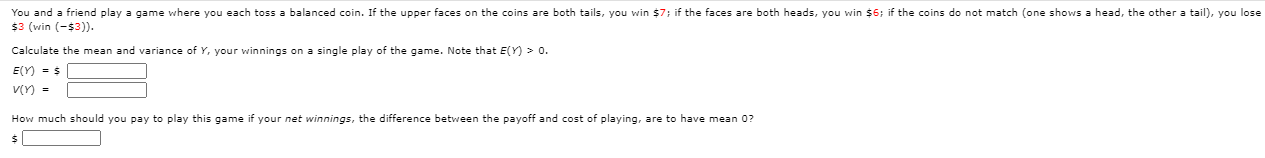# Question Solved1 AnswerYou and a friend play a game where you each toss a balanced coin. If the upper faces on the coins are both tails, you win \$7; if the faces are both heads, you win \$6; if the coins do not match (one shows a head, the other a tail), you lose \$3 (win (-\$3)). Calculate the mean and variance of y, your winnings on a single play of the game. Note that E(Y) > 0. EY) = V(Y) = How much should you pay to play this game if your net winnings, the difference between the payoff and cost of playing, are to have mean 0?Transcribed Image Text: You and a friend play a game where you each toss a balanced coin. If the upper faces on the coins are both tails, you win \$7; if the faces are both heads, you win \$6; if the coins do not match (one shows a head, the other a tail), you lose \$3 (win (-\$3)). Calculate the mean and variance of y, your winnings on a single play of the game. Note that E(Y) > 0. EY) = V(Y) = How much should you pay to play this game if your net winnings, the difference between the payoff and cost of playing, are to have mean 0?
More
Transcribed Image Text: You and a friend play a game where you each toss a balanced coin. If the upper faces on the coins are both tails, you win \$7; if the faces are both heads, you win \$6; if the coins do not match (one shows a head, the other a tail), you lose \$3 (win (-\$3)). Calculate the mean and variance of y, your winnings on a single play of the game. Note that E(Y) > 0. EY) = V(Y) = How much should you pay to play this game if your net winnings, the difference between the payoff and cost of playing, are to have mean 0?